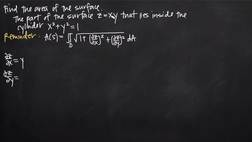PLAY PREVIEW

### Segments in this Video

#### How to Find the Area of a Surface(03:53)

FREE PREVIEW

We're going to be using the formula for the area of a surface, A, to find the area of the part of the surface.

#### Converting Polar Coordinates(01:56)

When we simplify, there are three conversion formulas we use.

#### Solving for DR(01:27)

With this problem, rdr is inside of the integral. We will take the integral and we will evaluate this on the interval 0 to 1.

#### Evaluating the Integral Equation(02:20)

We're trying to find the equation of the curve that goes through the points (1, 2) and (0, 0).We can start by writing the equation of the line that goes through those two points.

#### Theta and Area of Surface S(01:13)

This is our final answer, which is the value of the area of the surface S when the surface S is the part of z equals xy that lies inside the cylinder with this equation.

#### Credits(00:00)

Credits

For additional digital leasing and purchase options contact a media consultant at 800-257-5126
(press option 3) or sales@films.com.

# Area of a surface

Part of the Series : Integral Calc: Calculus 3
 3-Year Streaming Price: \$49.95

Share

### Description

This video tutorial works through math problems/equations that address topics in Calculus 3, Vectors. This specific tutorial addresses Area of a surface.

Length: 11 minutes

Item#: BVL275653Closed Captioned